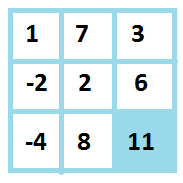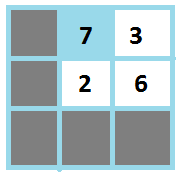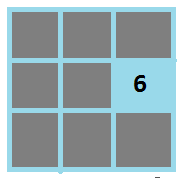Xsquare and A Gaming Matrix
Tag(s):

## Algorithms, Dynamic Programming, Hard

Problem
Editorial
Analytics

Xsquare loves to play games very much. Today, he has a special square matrix M of size N x N containing both non-negative as well as negative integers which he calls a Gaming Matrix. Rows and columns of his Gaming Matrix are numbered from 1 to N.

In one move, he performs following operations :

• He can select any out of the four available corner cells of his gaming matrix.
• Add value of the selected cell to his score.
• If N > 1, Replace existing gaming matrix with any of available square matrix of size N-1.

NOTE : For a gaming matrix of size N x N where N > 1, Xsquare can select any square matrix of size N-1 as his new gaming matrix which does not contains the discarded cell.

• If N == 1, Game is over.

Refer to the figure for better understanding of a gaming move.

Let us consider the following Gaming Matrix of size 3 x 3.• During his first move, Xsquare selected the highlighted cell and added its value to his score.
• Xsquare then discarded this selected cell.• Xsquare selected the highlighted square matrix as new Gaming Matrix ( offcourse it is of size N-1 i.e 2 x 2 ).
• During his second move, Xsquare selected the highlighted cell and added its value to his score.
• Xsquare then discarded this selected cell.• Finally during his third move, Xsquare selected the highlighted cell i.e cell with value 6.
• As N == 1, Game is over.

This way Xsquare managed to get a score 24 which is maximum possible score .

Note that Xsquare cannot leave the game in between till it is over.

Your task is very very simple. Given a Gaming Matrix of size N x N. Find the maximum possible score that can be achieved following the above moves .

### Input

First line of input contains a single integer T denoting the number of test cases. First line of each test case contains a single integer N denoting the size of the matrix M. Next N line of each test cases contains N space separated integers where jth element in the ith line denotes the value of the cell M[i][j].

### Output

For each test case, Print the maximum score that can be achieved from the given gaming matrix .

### Constraints

1 <= T <= 50

1 <= N <= 100

-109 <= M[i][j] <= 109

### Scoring

Subtask 1 : 1 <= T <= 50 , 1 <= N <= 32 (40 pts)

Subtask 2 : 1 <= T <= 50 , 1 <= N <= 100 (60 pts)

*Problem statement in native language : http://hck.re/z5lNOe *

SAMPLE INPUT
1
3
1 7 3
-2 2 6
-4 8 11

SAMPLE OUTPUT
24


Time Limit: 3.0 sec(s) for each input file.
Memory Limit: 256 MB
Source Limit: 1024 KB
Marking Scheme: Marks are awarded when all the testcases pass.
Allowed Languages: Bash, C, C++, C++14, Clojure, C#, D, Erlang, F#, Go, Groovy, Haskell, Java, Java 8, JavaScript(Rhino), JavaScript(Node.js), Julia, Kotlin, Lisp, Lisp (SBCL), Lua, Objective-C, OCaml, Octave, Pascal, Perl, PHP, Python, Python 3, R(RScript), Racket, Ruby, Rust, Scala, Swift, Swift-4.1, TypeScript, Visual Basic

## CODE EDITOR

Initializing Code Editor...

## This Problem was Asked inChallenge Name

Japan National Programming Challenge

OTHER PROBLEMS OF THIS CHALLENGE
• Math > Basic Math
• Algorithms > Searching
• Math > Linear Algebra